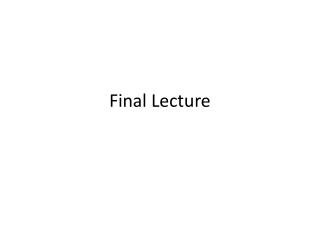DownloadDownload PresentationFinal Lecture

# Final Lecture

Download Presentation## Final Lecture

- - - - - - - - - - - - - - - - - - - - - - - - - - - E N D - - - - - - - - - - - - - - - - - - - - - - - - - - -
##### Presentation Transcript

1. Final Lecture

2. Instructions in Exam • ANSWER 2 QUESTIONS. • There will be a choice of 3 questions. • Each question will be in parts e.g. a, b, c. • You can see the marks awarded for correct answers for each part.

3. Tips • Before Exam, try past exam papers under exam conditions • (e.g. turn off your phone and see if you can answer an exam paper comfortably within the time). • Read all of the questions. • Sketch out your answers. • Keep track of time e.g. spend about half the time on each question. • You do not need to cheat (e.g. take in formula). • Any reasoning you do with diagrams, I would do in pencil first, • mistakes can be rubber out (and you can write in pen later). • This is an easy exam so relax and do not worry. • WRITE NEATLY

4. Time and Place • 04:30PM-06:30PM, 14/01/2008 • Algorithmic Problem Solving • Year 2 CS; Year 2 CSM • SSB302

5. a≡b verse a=b • With just 2 variable these are the same. • With 3 or more variables they are different. • a=b=c (read conjunctively) mean they are all the same value • just like integers or reals in maths. • a≡b ≡c (read associately) i.e. a≡(b ≡c ) or as (a≡b) ≡c • but actually these are both the same so we can forget about the () • but a=b=c is not the same as a≡b ≡c

6. Knight and Knaves - again :( • Knight tell truth, Knaves lie. • (let 1 be Knights 0 be Knaves) • If we ask A a Yes/No Question Q, the response to the question will be true in 2 cases • 1. the question is true and A is a Knight • 2. the question is false and A is a Knave • (in the other 2 cases it is false) • We can summarize this as Q=A • where Q is a yes/no question (i.e. a Boolean proposition) • and A is the Boolean proposition "A is a knight"

7. Question to ask • We want to find if A and B are the same type (for example). • The required response is A=B (i.e. A and B are the same type) • From the previous slide we know Q=A, • therefore (Q=A)=(A =B), • what does this simplify to?

8. Mex Numbers (again) • draw a graph (random) and label with letters. • How is a state described. • Now label mex numbers • A mex number is the smallest natural number not in the mex number of the successors.

9. Mex Numbers in Sum Games • In a matchstick game we can remove 1 or 2 matches, label the diagram with a pattern. • What it the mex number of the nth position? • How do we play the sum game? • How do we play a pair of matchstick games? • How about a random graph and a the component game from coursework 2.

10. Fuse Clocks – show fuseclock.ps file • Given two fuses which burn for m and n, create as many clocks as possible • Clearly m and n need to be different.

11. Before the exam. • Seminars. • If you have problem, mail me at • John.woodward@nottingham.edu.cn • John.r.woodward@gmail.com • Good luck in Exam.